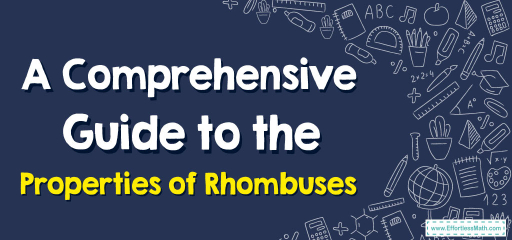# A Comprehensive Guide to the Properties of Rhombuses

A rhombus, often viewed as a "slanted square," is a captivating quadrilateral that brings with it a set of distinctive properties. Every side of a rhombus is of equal length, making it a special type of parallelogram. While it shares some features with other parallelograms, its equilateral nature and intriguing internal angles make it a standout figure in geometry. As we delve deeper, we'll discover the rich geometric attributes and characteristics that make rhombuses both fascinating and fundamental in the realm of shapes.## Step-by-step Guide: Properties of The Rhombus

Definition of a Rhombus:
A rhombus is a four-sided polygon (quadrilateral) where all four sides are of equal length. This ensures that opposite angles of a rhombus are equal.

Parallel Opposite Sides:
The opposite sides of a rhombus are always parallel to each other, making it a type of parallelogram.

Equal Diagonals:
While the diagonals of a rhombus are not necessarily equal in length, they do bisect each other at right angles. This means that they divide each other into two equal parts.

Area:
The area of a rhombus can be determined using its diagonals. If $$d_1$$ and $$d_2$$ represent the lengths of the diagonals, then the area $$A$$ is given by:
$$A = \frac{d_1 \times d_2}{2}$$

Perimeter:
As all sides of a rhombus are equal, if a side is of length $$s$$, the perimeter $$P$$ is:
$$P = 4s$$

Acute and Obtuse Angles:
A rhombus can have both acute and obtuse angles. The opposite angles are always equal. If one angle is acute, the angle adjacent to it will be obtuse, and vice versa.

### Examples

Example 1:
Finding the Area of a Rhombus.
Given: A rhombus with diagonals measuring $$10$$ units (longer diagonal) and $$8$$ units (shorter diagonal).

Solution:
The area of a rhombus can be determined using the formula:
$$A = \frac{d_1 \times d_2}{2}$$
Where $$d_1$$ and $$d_2$$ are the lengths of the diagonals. Essentially, the area is half the product of its diagonals. This formula stems from the fact that the diagonals of a rhombus are perpendicular bisectors of each other, dividing the rhombus into four congruent right triangles.
Area: Using the formula, we get:
$$A = \frac{d_1 \times d_2}{2} = \frac{10 \times 8}{2} = 40$$ square

Example 2:
Calculating the Perimeter of a Rhombus.
Given: A rhombus with a side length of $$7$$ units.

Solution:
The perimeter of a rhombus, or any polygon for that matter, is simply the sum of the lengths of its sides. Since all sides of a rhombus are of equal length, the perimeter can be calculated by multiplying one side by four.
Perimeter: Using the concept above, we find:
$$P = 4 \times \text{side length} = 4 \times 7 = 28$$ units

### Practice Questions:

1. A rhombus has diagonals measuring $$12$$ units and $$16$$ units. Compute its area.
2. If one side of a rhombus is $$9$$ units long, determine its perimeter.
3. One diagonal of a rhombus is half the length of the other. If the longer diagonal is $$20$$ units, find the area of the rhombus.

1. Area: $$A = \frac{12 \times 16}{2} = 96$$ square units.
2. Perimeter: $$P = 4 \times 9 = 36$$ units.
3. The shorter diagonal is $$10$$ units. Area: $$A = \frac{20 \times 10}{2} = 100$$ square units.

### What people say about "A Comprehensive Guide to the Properties of Rhombuses - Effortless Math: We Help Students Learn to LOVE Mathematics"?

No one replied yet.

X
30% OFF

Limited time only!

Save Over 30%

SAVE $5 It was$16.99 now it is \$11.99